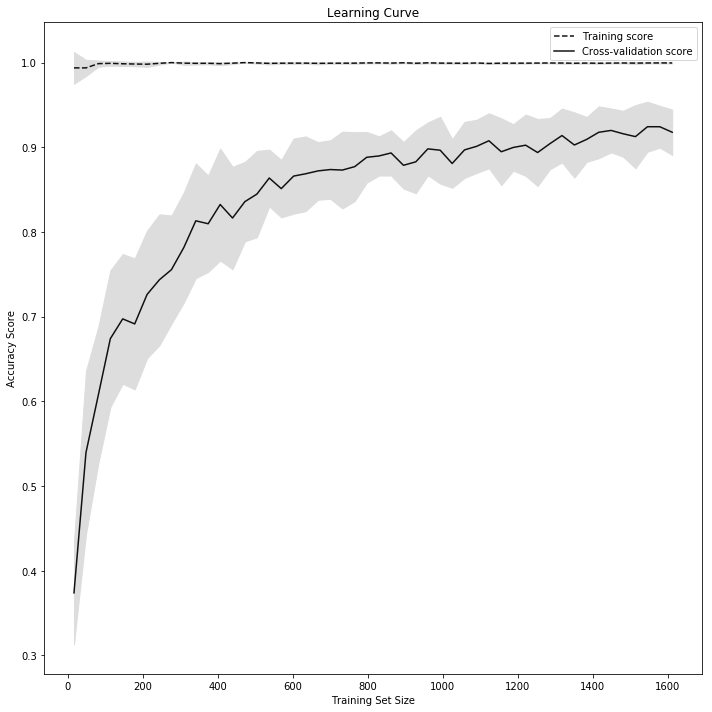How to plot a learning Curve in Python?
0

# How to plot a learning Curve in Python?

This recipe helps you plot a learning Curve in Python
In :
```## How to plot a learning Curve in Python
def Snippet_139():
print()
print(format('How to plot a learning Curve in Python','*^82'))

import warnings
warnings.filterwarnings("ignore")

import numpy as np
import matplotlib.pyplot as plt
from sklearn.ensemble import RandomForestClassifier
from sklearn.model_selection import learning_curve

# Create feature matrix and target vector
X, y = digits.data, digits.target

# Plot Learning Curve
# Create CV training and test scores for various training set sizes
train_sizes, train_scores, test_scores = learning_curve(RandomForestClassifier(),
X, y, cv=10, scoring='accuracy', n_jobs=-1,
# 50 different sizes of the training set
train_sizes=np.linspace(0.01, 1.0, 50))

# Create means and standard deviations of training set scores
train_mean = np.mean(train_scores, axis=1)
train_std = np.std(train_scores, axis=1)

# Create means and standard deviations of test set scores
test_mean = np.mean(test_scores, axis=1)
test_std = np.std(test_scores, axis=1)

# Draw lines
plt.subplots(1, figsize=(10,10))
plt.plot(train_sizes, train_mean, '--', color="#111111",  label="Training score")
plt.plot(train_sizes, test_mean, color="#111111", label="Cross-validation score")

# Draw bands
plt.fill_between(train_sizes, train_mean - train_std, train_mean + train_std, color="#DDDDDD")
plt.fill_between(train_sizes, test_mean - test_std, test_mean + test_std, color="#DDDDDD")

# Create plot
plt.title("Learning Curve")
plt.xlabel("Training Set Size"), plt.ylabel("Accuracy Score"), plt.legend(loc="best")
plt.tight_layout(); plt.show()

Snippet_139()
```
```**********************How to plot a learning Curve in Python**********************
```In [ ]:
```
```